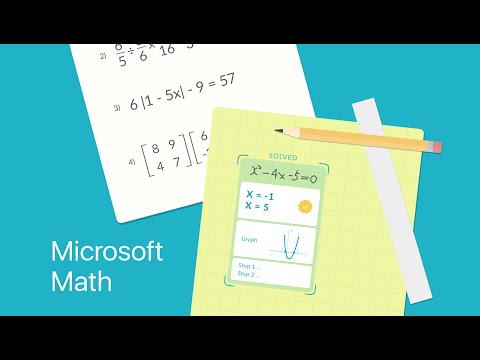Microsoft Math solver app provides help with a variety of problems including arithmetic, algebra, trigonometry, calculus, statistics, and other topics using an advanced AI powered math solver. Simply write a math problem on screen or use the camera to snap a math photo. Microsoft Math problem solver instantly recognizes the problem and helps you to solve it with â¡FREE Step-By-Step Explanationsâ¡, interactive graphs, similar problems from the web and online video lectures. Quickly look up related math concepts. Get help with your homework problems and gain confidence in mastering the techniques ð¯ with Microsoft Math. â¡It is absolutely FREE and No Ads!â¡

## HIGHLIGHTS

â âï¸ Write a math equation on screen as you naturally do on paper
â ð· Scan printed or handwritten math photo
â â¨ï¸ Type and edit using advanced scientific math calculator
â Get interactive Step-by-Step explanations ð¡ & Graphing calculator ð
â Import images with math equations from gallery ð¼ï¸
â Scan and Solve Math Worksheets with multiple problems
â ðSearch the web for similar problems and video lectures
â Try math word problems
â Scan and plot x-y data tables for linear/non-linear functions
â Learn math in your language - supports Chinese, French, German, Hindi, Italian, Japanese, Portuguese, Russian, Spanish, and many more

## SUPPORTED PROBLEMS

â Elementary: arithmetic, real, complex numbers, LCM, GCD, factors, roman numerals
â Pre-Algebra: radicals and exponents, fractions, matrices, determinants
â Algebra: quadratic equations, system of equations, inequalities, rational expressions, linear, quadratic and exponential graphs
â Word problems on math concepts, number theory, probability, volume, surface area
â Basic Calculus: Summations, Limits, derivatives, integrals
â Statistics: Mean, Median, Mode, Standard Deviation, permutations, combinations

Find out more about Microsoft Math Solver app on our website ð Link: https://math.microsoft.com